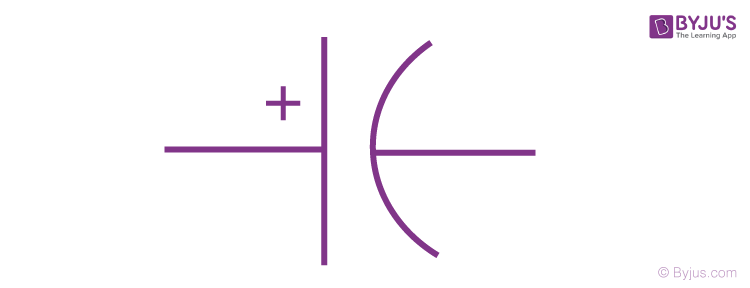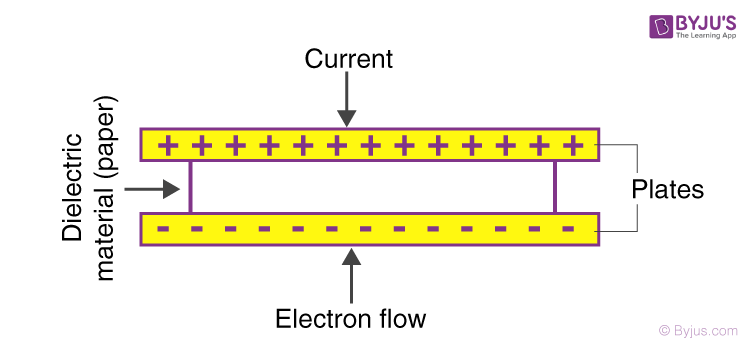# Capacitor Types

As we know capacitor is one of the basic components used in an electrical circuit like resistors, inductors, and many more. The capacitor is a passive device that is available in a wide variety. They are classified based on various aspects. Let us know the detailed classification of capacitors along with capacitor types.

## What Is a Capacitor?

• A capacitor is defined as a passive component which is used for storing electrical energy. A capacitor is made of two conductors that are separated by the dielectric material. These dielectric materials are in the form of plates which can accumulate charges.
• One plate is for a positive charge while the other is for a negative charge.
• Capacitance is the effect of the capacitor. Capacitance is defined as the ratio of electric charge Q to the voltage V and it is expressed as
 C = Q/V

Where,

• Q is the electric charge measured in coulombs
• C is the capacitance measured in farad
• V is the voltage across the plates measured in volts

## How Are Capacitors Classified?

According to structure, capacitors are classified as:

• Fixed Capacitors
• Variable Capacitors
• Trimmer Capacitors

The capacitors are classified into two types according to polarization:

• Polarized
• Unpolarized
• A polarized capacitor is an important electronic circuit component and is often termed an electrolytic capacitor. These capacitors are used to achieve high capacitive density.
• Unpolarized capacitors are preferred over polarized capacitors because it doesn’t get destroyed by reverse voltage and can be used in pure AC circuits. They also find applications in DC circuits as they don’t have positive and negative ends. The frequency of the unpolarized capacitor is high, and the leakage current is low. After understanding the classification of capacitors, let us learn about capacitor types.

## Types of Capacitors

Let us now know various types of capacitors. Capacitors are categorized into 2 mechanical groups. Fixed Capacitors consist of fixed capacitance value and variable capacitance with variable capacitance value. Beneath are a brief description of various capacitor types and their properties.

• Ceramic Capacitors
• Film Capacitors
• Power Film Capacitors
• Electrolytic Capacitors
• Ceramic capacitors
• Film capacitors
• Paper Capacitors
• Electrolytic capacitors

## Ceramic Capacitors

A ceramic capacitor is considered to be one of the most commonly used capacitors. The material used in this capacitor type is dielectric. Also, ceramic capacitors are non-polar devices which means that they can be used in any direction in the circuit.

#### Ceramic Capacitor SymbolDepending on the availability of the capacitor, ceramic capacitors are classified into three groups:

• Surface mount multi-layered ceramic capacitors
• Microwave bare lead-less disc ceramic capacitors

Depending on the temperature range, temperature drift, and tolerance, ceramic capacitors are classified into the following classes:

• Class 1 ceramic capacitors: These capacitors are considered to be the most stable capacitors with linear characteristics.
• Class 2 ceramic capacitors: These capacitors perform better for volumetric efficiency but their accuracy and stability are at stake. They find applications in coupling and decoupling.
• Class 3 ceramic capacitors: These capacitors have high volumetric efficiency with low accuracy and low dissipation factor. They are used in decoupling.

## Applications of Ceramic Capacitors

• Ceramic capacitors are used in printed circuit boards that are used in high-density applications.
• Their non-polarity makes them suitable for general usage.
• They find applications in DC motors as they are used for reducing the RF noise.
• Ceramic capacitors are used in transmitter stations where resonant circuits are used.

## Film Capacitors

Film capacitors are also known as a polymer film, plastic film, or film dielectric. The advantage of film capacitors is that they are inexpensive and come with limitless shelf life. The film capacitor uses a thin dielectric material with the other side of the capacitor metalized. Depending on the application, the film capacitor is rolled into thin films. The general voltage range of these capacitors is from 50 V to 2 kV.

### Types of Film Capacitors

Depending on the dielectric material used and applications, the following is the classification of the film capacitor:

• Heavy-duty snubber capacitors
• SMD style capacitors
• Axial style capacitors

### Applications of Film Capacitors

• These capacitors are used as safety capacitors and in electromagnetic interference.
• Power film capacitors find applications in power electronics.
• These capacitors are used for safeguarding the devices from sudden voltage spikes.
• Film capacitors are used for improving the power factor of the device.

Related Articles:

### Power Film Capacitors

Construction techniques and materials that are used in power film capacitors are similar to that of ordinary film capacitors. Polypropylene film is used as a dielectric in a capacitor.

### Electrolytic Capacitors

In an electrolytic capacitor metallic anode coated with an oxidized layer used as a dielectric. These capacitors are polarized. Electrolytic capacitors are categorized based on their dielectric.

• Aluminum electrolytic capacitors – aluminum oxide (dielectric).
• Tantalum electrolytic capacitors – tantalum pentoxide (dielectric).
• Niobium electrolytic capacitors – niobium pentoxide (dielectric).

### Electrolytic Capacitor Symbol### Applications of Electrolytic Capacitors

• Electrolytic capacitors are used when there is a requirement for large capacitance.
• They are used as filtering devices that lower the ripple voltage.
• They are used in audio amplifiers to reduce the electrical noise that is induced by the main supply.
• Electrolytic capacitors are used in smoothing the input and output signals in a DC signal that has a weak AC component.

## Accelerate Your JEE Main & Advanced Exam Preparation by Watching this Video about Magnetism and Force on Dielectric in Charged Capacitor## Paper Capacitor

Paper capacitor is also known as a fixed capacitor in which paper is used as the dielectric material. The amount of electric charge stored by the paper capacitor is fixed. It consists of two metallic plates, and paper, which is used as a dielectric material, is placed between these plates.

#### Paper Capacitor Symbol### Applications of Paper Capacitor

• These capacitors are used in noise filtering, coupling, and decoupling systems.
• They are also used for blocking the DC signals so that AC signals are passed through.
• Sensors such as humidity sensors, fuel level sensors, etc used paper capacitors.
• Paper capacitors are used in audio systems of cars as they provide extra power to the amplifiers.

## What Are Common Capacitors?

Capacitors are mainly divided into two mechanical groups:

• Fixed capacitors
• Variable capacitors

Some common capacitors and their names are mentioned in the table below.

 Ceramic capacitors Aluminum, tantalum, and niobium electrolytic capacitors Film and paper capacitors Polymer capacitors Silver mica, glass, silicon, air-gap, and vacuum capacitors Double-layer capacitors Pseudo capacitors Hybrid capacitors

### Values of Capacitor

Knowing the capacitor types, and applications, let us now learn the value of capacitor. Depending on the type of capacitor, the values of the capacitor vary. For example, electrolytic capacitors have their values printed on their body along with the pins. Disc capacitors have their values represented in terms of PF, uF, KPF, etc. a black band is used to represent the negative terminal of the capacitor.

Therefore, the following are the ways in which capacitors values could be read:

• The capacitance value of the capacitor is expressed in picofarads.
• If a capacitor has the third number as zero, then the value is expressed as P.
• If a capacitor has 3 digits, then the third number represents the number of zeros of that capacitor. This is usually after the second number.
• The conversion of values to KPF or uF becomes easy if the value is in PF.

## Watch the Video below to Learn Solved Problems on Capacitors## Frequently Asked Questions – FAQs

Q1

### Name the metal that is used in the capacitor.

Metals such as aluminium, silver, and other metals are used in the construction of the plates of the capacitors. The dielectric materials which are used between these metallic plates are paper, ceramic, or rubber, depending on the application of the capacitor.

Q2

### How to analyze the quality of the capacitors?

The quality of the capacitors is analyzed using a digital multimeter. The multimeter is connected to the ends of the capacitor plates. The positive end of the capacitor is connected to the red lead, while the opposing end of the capacitor is connected to the black lead of the multimeter. If the reading goes from zero to infinity, the capacitor is considered in good condition.

Q3

### Differentiate between a start capacitor and a run capacitor.

The start capacitor is used when the current to voltage lag is required, whereas the run capacitor is used for enhancing the current supply.

Q4

### What is the polarity of the film capacitors?

The film capacitors do not have polarity as they are non-polarized.

Q5

### What is the principle of the capacitor?

The principle of a capacitor is based on an insulated conductor whose capacitance is increased gradually when an uncharged conductor is placed next to it.

Hope you have learned about various types of capacitors. Stay tuned with BYJU’S to know about various science and math concepts.

Test your knowledge on Capacitor types